# Given a true PRG G, show whether or not the following functions are necessarily PRGs. If true, pr...

Given a true PRG G, show whether or not the following functions

are necessarily PRGs. If true, prove it. If not give a counterexample. Note that jj denotes

concatenation

G'(s) : = G (s) II G (s    1 IsI  )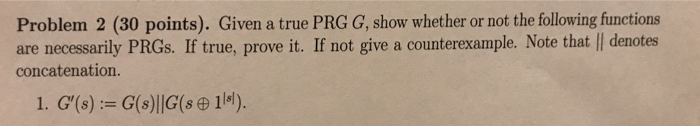Problem 2 (30 points). Given a true PRG G, show whether or not the following functions are necessarily PRGs. If true, prove it. If not give a counterexample. Note that l| denotes concatenation.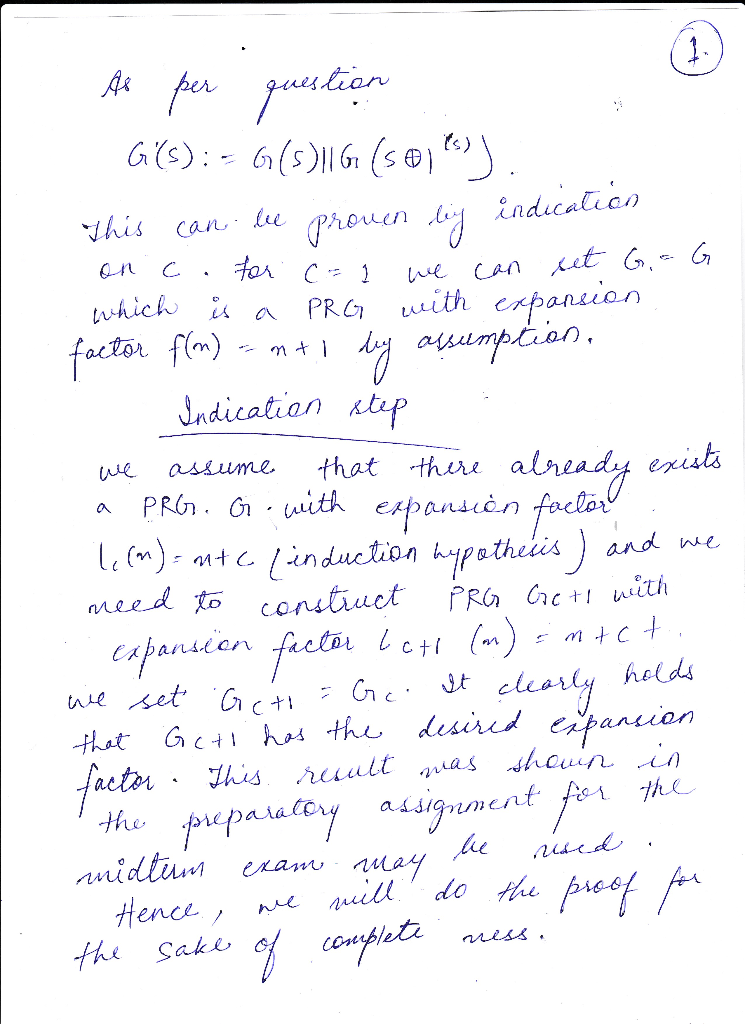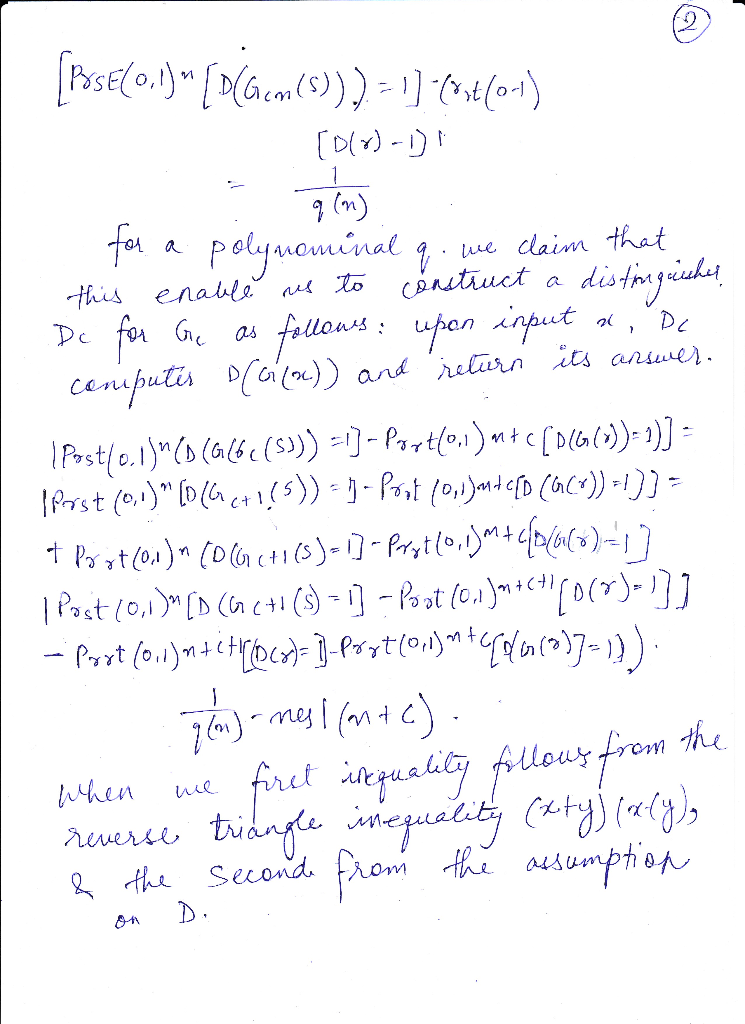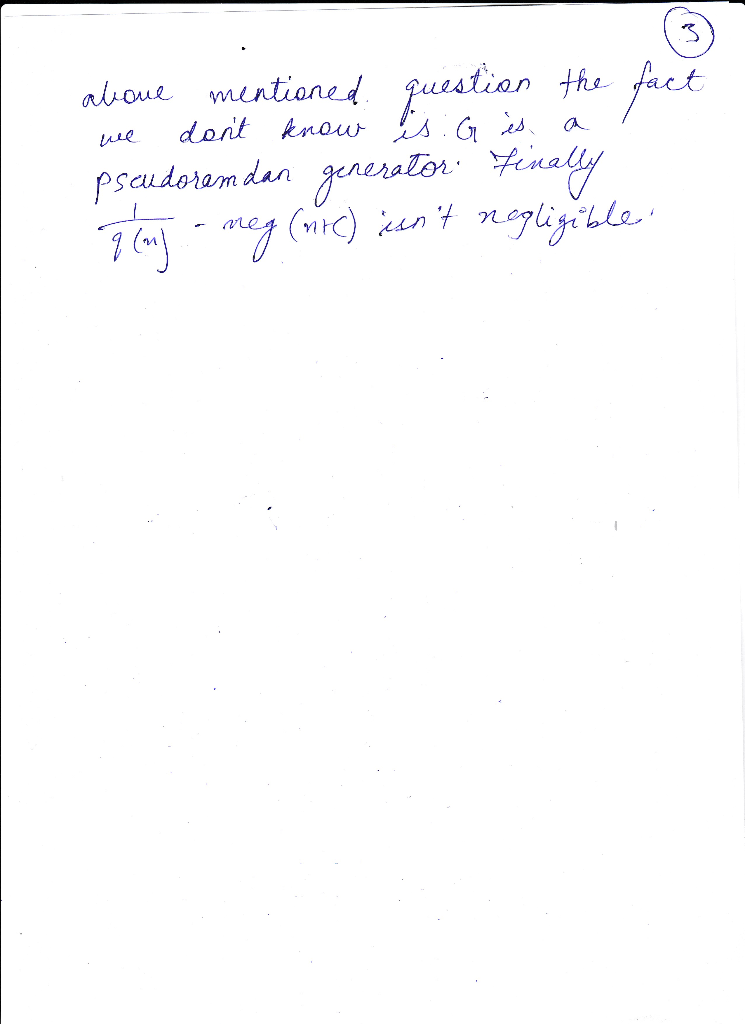#### Earn Coin

Coins can be redeemed for fabulous gifts.

Similar Homework Help Questions
• ### Problem 2 (30 points). Given a true PRG G, show whether or not the following functions are necess...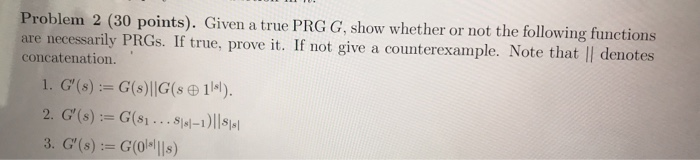Problem 2 (30 points). Given a true PRG G, show whether or not the following functions are necessarily PRGs. If true, prove it. If not give a counterexample. Note that || denotes concatenation Problem 2 (30 points). Given a true PRG G, show whether or not the following functions are necessarily PRGs. If true, prove it. If not give a counterexample. Note that || denotes concatenation

• ### Problem 1: Determine whether the statement is true or false. If the statement is true, then...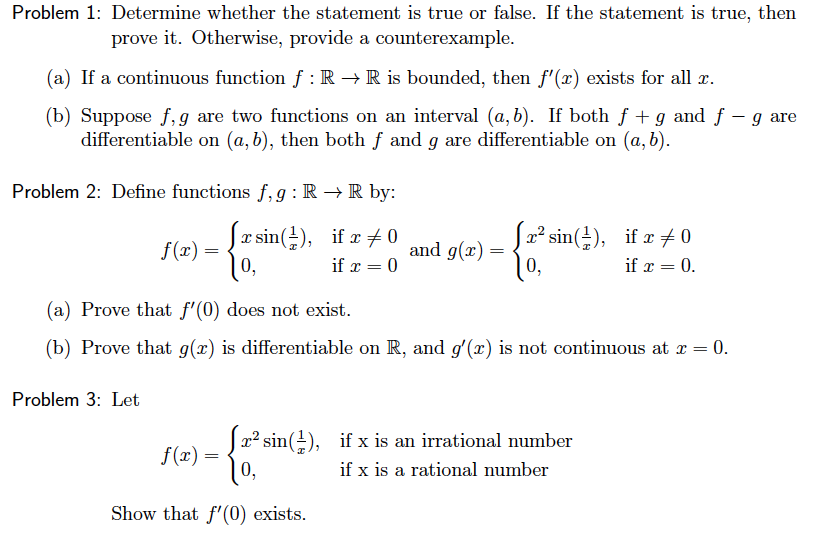Problem 1: Determine whether the statement is true or false. If the statement is true, then prove it. Otherwise, provide a counterexample. (a) If a continuous function f:R +R is bounded, then f'(2) exists for all x. (b) Suppose f.g are two functions on an interval (a, b). If both f + g and f - g are differentiable on (a, b), then both f and g are differentiable on (a,b). Problem 2: Define functions f,g: RR by: x sin(-),...

• ### Determine whether the statement is true or false. If false, explain why or give a counterexample that shows it is false. (2 pts each) b. If f(x,y) S g(x, y) for all (x, y) in , and both f and g are...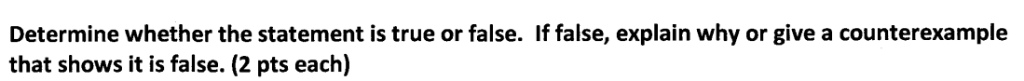Determine whether the statement is true or false. If false, explain why or give a counterexample that shows it is false. (2 pts each) b. If f(x,y) S g(x, y) for all (x, y) in , and both f and g are continuous over 2, then c. If f is continuous over 2 and 22, and if JJ, dA- jJa,dA, then f(x.y) dA- Jf(x.y) dA for any function fx,y). Determine whether the statement is true or false. If false, explain...

• ### Let G be a pseudorandon generator with expansion factor l(n) > 2n. In each of the...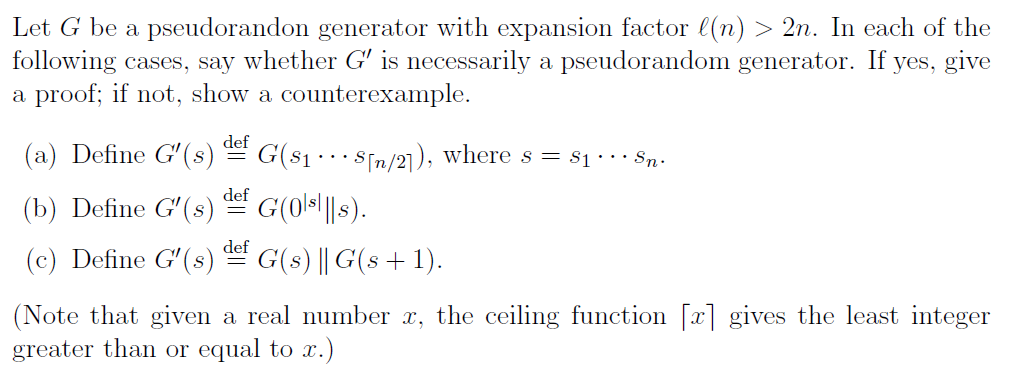Let G be a pseudorandon generator with expansion factor l(n) > 2n. In each of the following cases, say whether G' is necessarily a pseudorandom generator. If yes, give a proof; if not, show a counterexample. def def (a) Define G'(s) G(\$1.5[n/21), where s = \$1... Sn. (b) Define G'(s) G(018|||s). (c) Define G'(s) G(s) || G(s + 1). (Note that given a real number x, the ceiling function [x] gives the least integer greater than or equal to x.)...

• ### 1. Let f and g be functions with the same domain and codomain (let A be...

1. Let f and g be functions with the same domain and codomain (let A be the domain and B be the codomain). Consider the following ordered triple h = (A, B, f LaTeX: \cap ∩ g) (Note: The f and g in the triple refer to the "rules" associated with the functions f and g). Prove that h is a function. Would the same thing be true if, instead of intersection, we had a union? If your answer is...

• ### how do u do 6? F-'(C-D)= F-'(C)-F-'(D). 4. (10 points) In following questions a function f...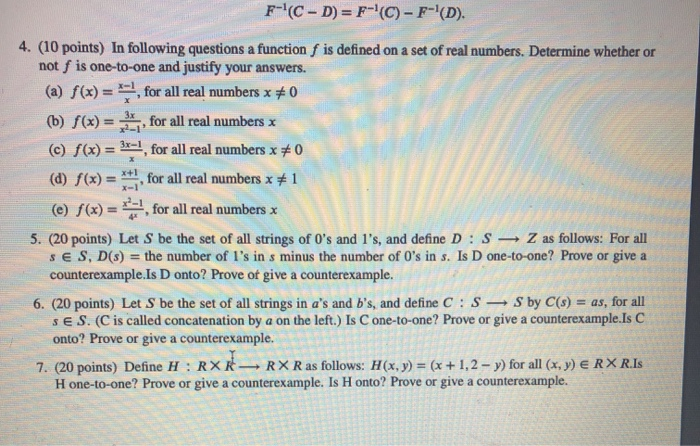how do u do 6? F-'(C-D)= F-'(C)-F-'(D). 4. (10 points) In following questions a function f is defined on a set of real numbers. Determine whether or not f is one-to-one and justify your answers. (a) f(x) = **!, for all real numbers x #0 (6) f(x) = x, for all real numbers x (c) f(x) = 3x=!, for all real numbers x 70 (d) f(x) = **, for all real numbers x 1 (e) f(x) = for all real...

• ### 6. Determine whether each of the following is true or false (note: the statement is true...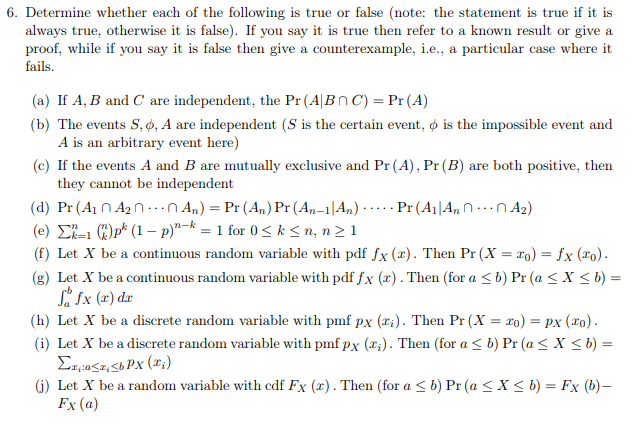6. Determine whether each of the following is true or false (note: the statement is true if it is always true, otherwise it is false). If you say it is true then refer to a known result or give a proof, while if you say it is false then give a counterexample, i.e., a particular case where it fails. (a) If A, B and C are independent, the Pr(AlBnc)- Pr (A) (b) The events S., A are independent (S is...

• ### 3. In this problem we consider only functions defined on the real numbers R A function f is close to a function g if r e Rs.t. Vy E R, A function f visits a function g when Vr E R, 3y E R s.t. Fo...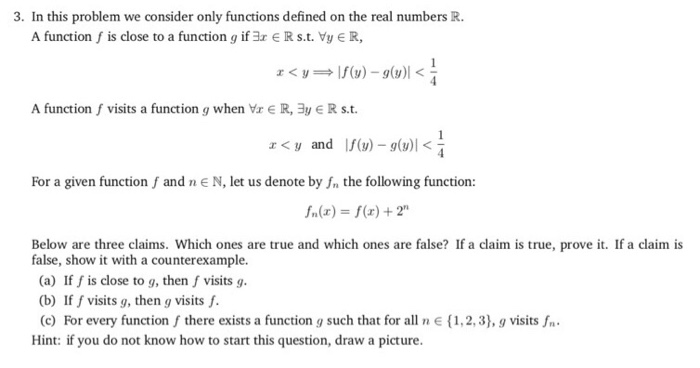3. In this problem we consider only functions defined on the real numbers R A function f is close to a function g if r e Rs.t. Vy E R, A function f visits a function g when Vr E R, 3y E R s.t. For a given function f and n E N, let us denote by fn the following function: Below are three claims. Which ones are true and which ones are false? If a claim is true,...

• ### Explain why or why not Determine whether the following state- ments are true and give an explanat...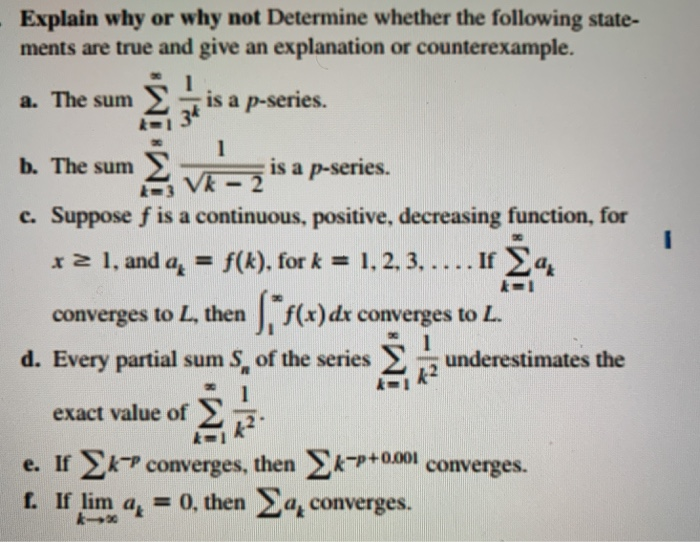any help would be awesome Explain why or why not Determine whether the following state- ments are true and give an explanation or counterexample. a. The sum Σ is a p-series. b. The sumeve IS a p-series. c. Suppose f is a continuous, positive, decreasing function, for re l'and ak =f(k), for k = 1,2,3, . . . . If Σ@g converges to L, then | f(x) dx converges to L. d. Every partial sums, of the series Σ underestimates...

• ### (a) Determine whether each of the following functions is uniformly continu- ous on the given domain....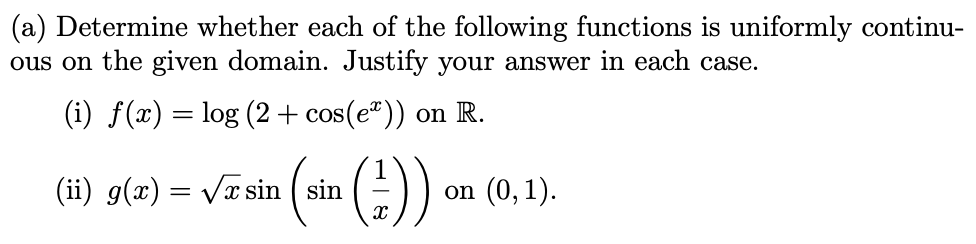(a) Determine whether each of the following functions is uniformly continu- ous on the given domain. Justify your answer in each case. (i) f(x) = log (2 + cos(e«)) on R. (ii) g(x) = Väsin ( sin on (0,1).